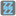• # How to post?

## Question related to missionFizz Buzz

hi guys,wrote a code:

def checkio(): print ("enter a number: ") number = input() number = int(number) if number % 3 == 0 and number % 5 == 0: print ('Fizz Buzz',number,'is divisible by 3 and 5') elif number % 3 == 0: print ('Fizz',number, 'is divisible by 3') elif number % 5 == 0: print ('Buzz',number,'is divisible by 5') else: print (number,",",number,"is not divisible by 3 or 5") checkio()

checkio()

but how do i post it for check?1